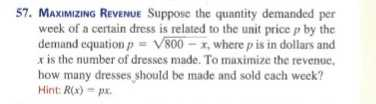### ¿Todavía tienes preguntas de matemáticas?

Pregunte a nuestros tutores expertos
Algebra
Pregunta57. Maximizing Revenue Suppose the quantity demanded per week of a certain dress is related to the unit price $$p$$ by the demand equation $$p = \sqrt { 800 - x }$$ , where $$p$$ is in dollars and

$$x$$ is the number of dresses made. To maximize the revenue, how many dresses should be made and sold cach week? Hint $$R ( x ) = p x$$ .

x=$$\frac{1600}{3}$$